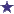# IV Math Calculation: How many MLs of reconstituted Penicillin per dose?

by Dominique
(USA )Syringe and Penicillin Vial

A manufacturer supplies penicillin in dry powder form that must be dissolved before use. A 20,000,000 unit vial of penicillin can be reconstituted with 32.4ml of sterile water to provide 500,000 units per ml. How many milliliters of the reconstituted vial should be used to make one bag containing 4 doses of 2,000,000 units per dose?

### Comments for IV Math Calculation: How many MLs of reconstituted Penicillin per dose?

Average RatingRatingAnswer: One IV bag/4 doses needs 16ML of the Penicillin Solution by: David 1ML = 500,000 units 2,000,000 units per dose would equal 4ML (4x500,000=2,000,000) 4MLx4 doses = 16ML